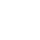## 蓄能器的选用

1. 选择方法

1) 最小压力P1和***压力P2－工作压力。

2) 可储存或利用的液体容积△V .

3) 方法和用途

4) 工作温度

5) 液体种类

6) 所需大流量

7) 使用场所

2.预充气压力

Po的极限值为：Po=0.9P1  Po min≥0.25×P2  Po max≤0.9P1

Ø 活塞蓄能器  Po=0.95-0.97 P1

Ø 脉动缓冲和减振Po=0.6-0.75 Pm或Po=0.8P1

Ø 液路缓冲

Po=0.6-0.9Pm

Ø 蓄能器+附加气瓶

Po=0.95-0.97P1

Po值适用于用户所要求的***工作温度。

Poc=Po3．计算原理

Po×Von=P1×V1n= P2×V2n

V1=压力P1时的氮气体积 P1（升）

V2=压力P2时的氮气体积 P2（升）

△V=所排放的或所储存的液体的容积（升）

Po=预充氮气压力（巴）

P1=最小工作压力（巴）

P2=***工作压力（巴）

n =多变指数

n = 1 如果氮气的压缩和膨胀发生的很慢，使得整个热交换过程位于气体和周围介质之间，这就是在恒温下；该状态为等温。

n = 1.4 如果运行速度进行的非常快，使得热交换没有发生；该状态为绝热。4 容积计算（等温）

Po×Vo=P1×V1= P2×V2

△V=V1－V2=Vo－VoVo=5 容积补偿（等温）

△V= VT×（T2－T1）×（β－3α）

VT =管道容积（升）

T2 =***温度（℃）

T1 =最小温度（℃）

β= 流体的体积膨胀系数（1/℃）

α= 管道线性膨胀系数（1/℃）

P1=最小允许工作压力（bar）

P2=***允许工作压力（bar）

Vo=6 泄漏补偿（等温）

ΔV=Q1×t

Po=0.9×P1

P1=最小允许工作压力（bar）

P2=***允许工作压力（bar）

Vo =7 容积计算（绝热）

Po×Von=P1×V1n= P2×V2n

ΔV = Vo=0.7143

Vo =VOT=VO；T2=t2(℃)+273=***工作温度（°K）

T1=t1(℃)+273=最小工作温度（°K）

VO=忽略了温度变化而计算出的容积（升）

VOT=考虑温度变化后的实际容积（升）

VOT=VO×Ci×（等温）

VOT=VO×Ca×（绝热）8紧急能量存储

Vo =ΔV = Von =1.4 绝热系数（快速排放阶段）

nc = 1-1.4多变系数（慢速排放阶段）

9 吸收脉动Vo =q=泵的工作容积（升）

=A×C(活塞面积×冲程)=Q/N（流率/冲程）

P=泵的平均工作压力（bar）

P1=P-X(bar)

P2=P+X(bar)

α=剩余脉动±（%）

K=此为系数，它与活塞的数量及泵的作用方式有关。

泵型号        K

1只活塞，单作用式  0.69

1只活塞，双作用式  0.29

2只活塞，单作用式  0.29

2只活塞，双作用式  0.17

3只活塞，单作用式  0.12

3只活塞，双作用式  0.07

4只活塞，单作用式  0.13

4只活塞，双作用式  0.07

5只活塞，单作用式  0.07

5只活塞，双作用式  0.023

6只活塞，单作用式  0.07

7只活塞，双作用式  0.023

10. 液压管线吸收冲击

ΔPmax(bar) =Vo=Vo= 蓄能器的容积 (升)

Q = 管路中的流量 (m3／h)

L = 管路的总长度（m）

γ = 液体的比重（Kg／m3）

V = 流速 (m/s)  =1000Q／3.6S

S = 管路的内截面积 (mm2)   =0.25×πd2

d = 管路内径(mm)

ΔP= 允许的超压(bar)

P1 = 自由流动的工作压力 (***压力，bar)

P2 = 允许的***压力 (***压力，bar)  = P1+ΔP

t = 减速时间(阀门关闭时间) (s)

11. 蓄能器＋附加气瓶（传送）

VOB =VOT －VOA

VOA = 蓄能器的容积

VOB = 附加气瓶的容积

• 取消“扫一扫”加入群聊gtag('config', 'AW-337502933'); "AW-337502933": { "groups": "default" }